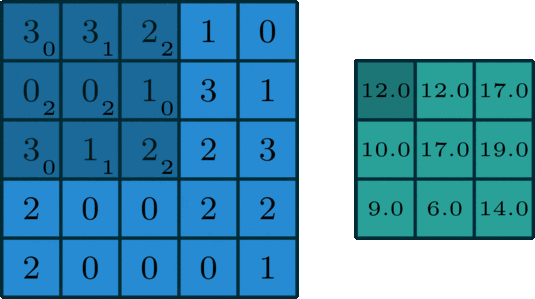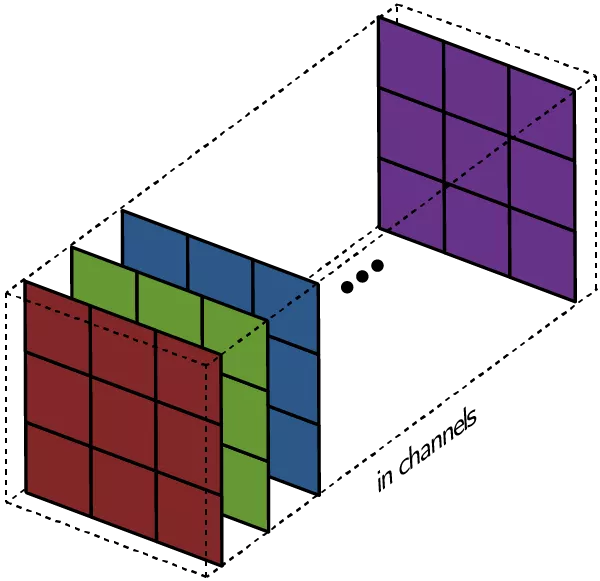# Pytorch 中 conv2d 处理的数据是什么样的？

## 对单通道数据进行卷积from IPython.core.interactiveshell import InteractiveShell
InteractiveShell.ast_node_interactivity = 'all' #显示多个变量结果
import numpy as np
import torch.nn as nn
import torch as t

#单通道是二维数据
img1 = np.array([[3,3,2,1,0],
[0,0,1,3,1],
[3,1,2,2,3],
[2,0,0,2,2],
[2,0,0,0,1]])

#5*5,说明是二维的
img1.shape
img1_tensor = t.Tensor(one_channel_data)
img1_tensor

(5, 5)

tensor([[3., 3., 2., 1., 0.],
[0., 0., 1., 3., 1.],
[3., 1., 2., 2., 3.],
[2., 0., 0., 2., 2.],

### 维度改变

• 将二维数据放到列表中，然后在将其转化为torch.Tensor
• 使用torch中的unsqueeze(0)方法,将维度增加一个维度

#### 列表法

img2 = np.array([[3,3,2,1,0],
[0,0,1,3,1],
[3,1,2,2,3],
[2,0,0,2,2],
[2,0,0,0,1]])
three_dims = [img2]
three_dims = t.Tensor(three_dims)
three_dims1.shape
three_dims1

torch.Size([1, 5, 5])

tensor([[[3., 3., 2., 1., 0.],
[0., 0., 1., 3., 1.],
[3., 1., 2., 2., 3.],
[2., 0., 0., 2., 2.],
[2., 0., 0., 0., 1.]]])

(1,5,5)元组中有三个数，所以我们成功将二维变成三维数据

#### unsqueeze(0)方法

img3 = np.array([[3,3,2,1,0],
[0,0,1,3,1],
[3,1,2,2,3],
[2,0,0,2,2],
[2,0,0,0,1]])

img3_tensor = t.Tensor(img3)
three_dims2 = img3_tensor.unsqueeze(0)
three_dims2.shape
three_dims2

torch.Size([1, 5, 5])

tensor([[[3., 3., 2., 1., 0.],
[0., 0., 1., 3., 1.],
[3., 1., 2., 2., 3.],
[2., 0., 0., 2., 2.],
[2., 0., 0., 0., 1.]]])

img3 = np.array([[3,3,2,1,0],
[0,0,1,3,1],
[3,1,2,2,3],
[2,0,0,2,2],
[2,0,0,0,1]])

img3_tensor = t.Tensor(img3)
four_dims = img3_tensor.unsqueeze(0).unsqueeze(0)
four_dims.shape
four_dims

torch.Size([1, 1, 5, 5])

tensor([[[[3., 3., 2., 1., 0.],
[0., 0., 1., 3., 1.],
[3., 1., 2., 2., 3.],
[2., 0., 0., 2., 2.],
[2., 0., 0., 0., 1.]]]])

(1, 1, 5, 5)元组中有四个数，所以我们成功将二维变成 四维数据

### 训练one_img = np.array([[3,3,2,1,0],
[0,0,1,3,1],
[3,1,2,2,3],
[2,0,0,2,2],
[2,0,0,0,1]])

#将二维数据转化为四维数据
one_data = t.Tensor(one_img).unsqueeze(0).unsqueeze(0)

#(1, 1, 5, 5) 成功转化为四维数据
one_data.shape

#卷积conv2d需要输入四维数据，其中输入和输出均为单通道，卷积核为3
conv = nn.Conv2d(in_channels=1, out_channels=1, kernel_size=3)

conv(one_data)

torch.Size([1, 1, 5, 5])

tensor([[[[-0.4410, -0.7628, -0.6724],
[-0.1663,  0.0914, -0.7396],
[ 0.5701, -0.1886, -0.2795]]]], grad_fn=<ThnnConv2DBackward>)

## 对多通道数据进行卷积from IPython.core.interactiveshell import InteractiveShell
InteractiveShell.ast_node_interactivity = 'all' #显示多个变量结果
import numpy as np
import torch.nn as nn
import torch as t

chanel_1 = [[3,3,2,1,0],
[0,0,1,3,1],
[3,1,2,2,3],
[2,0,0,2,2],
[2,0,0,0,1]]

chanel_2 = [[3,3,2,1,0],
[0,0,3,3,1],
[0,1,2,2,3],
[2,2,0,2,2],
[2,0,1,0,1]]

chanel_3 = [[3,3,2,1,0],
[0,0,3,3,1],
[3,3,2,2,3],
[2,2,0,2,2],
[2,5,0,0,1]]

#伪造一张照片，该照片是三通道数据
img_data2 = t.Tensor([chanel_1,
chanel_2,
chanel_3])

#三通道数据如果输入到conv2d中应该是四维数据。
img_data2 = img_data2.unsqueeze(0)
#(1, 1, 5, 5) 成功转化为四维数据
img_data2.shape

#卷积conv2d需要输入四维数据，其中输入为三通道in_channels=3，假设我们想输出单通道，则out_channels=1
conv = nn.Conv2d(in_channels=3, out_channels=1, kernel_size=3)

conv(img_data2)

torch.Size([1, 3, 5, 5])

tensor([[[[ 1.0329,  0.9904, -0.1885],
[ 0.0870,  0.2805,  0.3501],
[ 0.4509, -0.1977,  0.9282]]]], grad_fn=<ThnnConv2DBackward>)